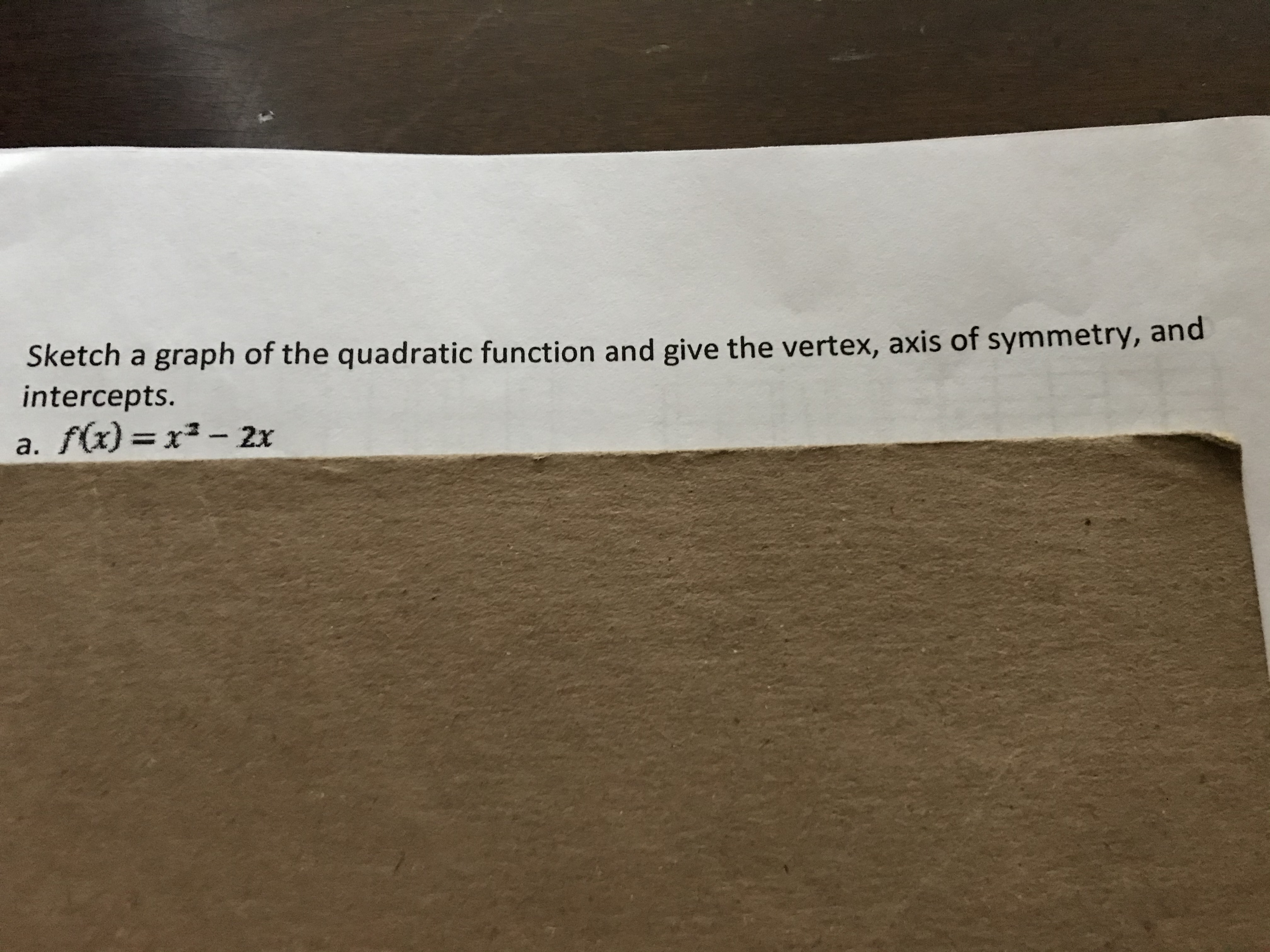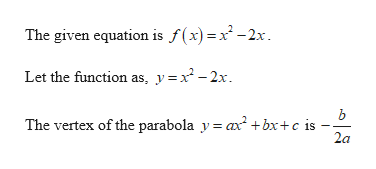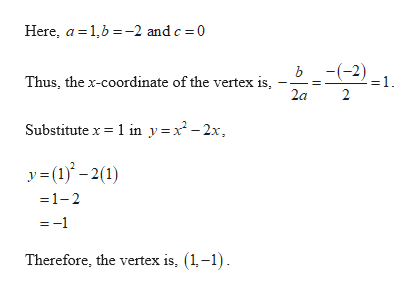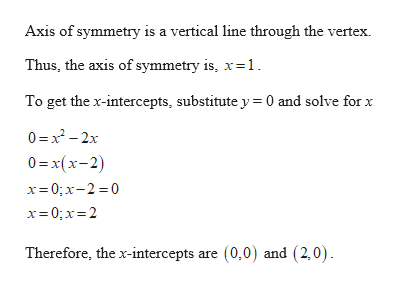# Sketch a graph of the quadratic function and give the vertex, axis of symmetry, andintercepts.a. f(x) =x²- 2x%3D

Question
1 views

how do i sketch the quadratic function below and how do i find the vertex , axis of symmetry, and intercepts ? step by step please.help_outlineImage TranscriptioncloseSketch a graph of the quadratic function and give the vertex, axis of symmetry, and intercepts. a. f(x) =x²- 2x %3D fullscreen
check_circle

Step 1help_outlineImage TranscriptioncloseThe given equation is f(x) =x -2x. Let the function as, y=x -2x. The vertex of the parabola y = ax +bx+c is - 2a fullscreen
Step 2help_outlineImage TranscriptioncloseHere, a = 1,6 =-2 and c = 0 ь - (-2). =1. Thus, the x-coordinate of the vertex is, 2a Substitute x = 1 in y=x² - 2x, y= (1)* – 2(1) =1-2 =-1 Therefore, the vertex is, (1,-1). || fullscreen
Step 3help_outlineImage TranscriptioncloseAxis of symmetry is a vertical line through the vertex. Thus, the axis of symmetry is, x=1. To get the x-intercepts, substitute y = 0 and solve for x 0 =x - 2x 0=x(x-2) x= 0; x-2 =0 x= 0; x=2 Therefore, the x-intercepts are (0,0) and (2,0). fullscreen

### Want to see the full answer?

See Solution

#### Want to see this answer and more?

Solutions are written by subject experts who are available 24/7. Questions are typically answered within 1 hour.*

See Solution
*Response times may vary by subject and question.
Tagged in